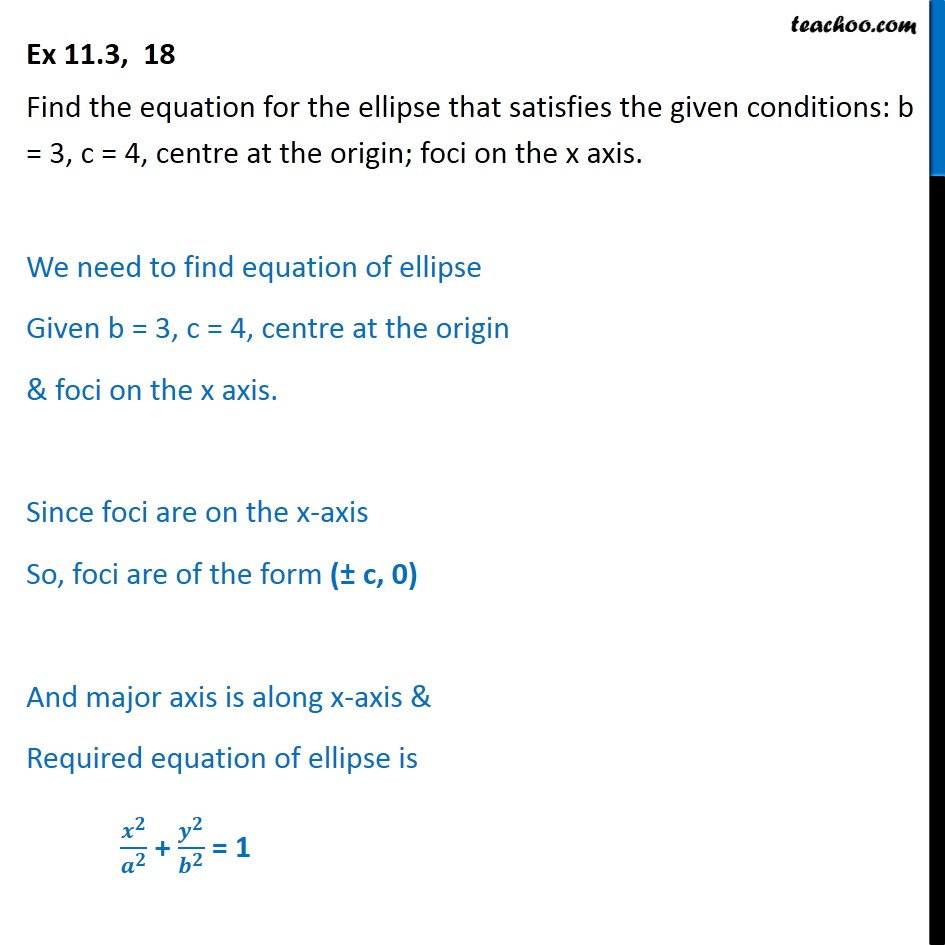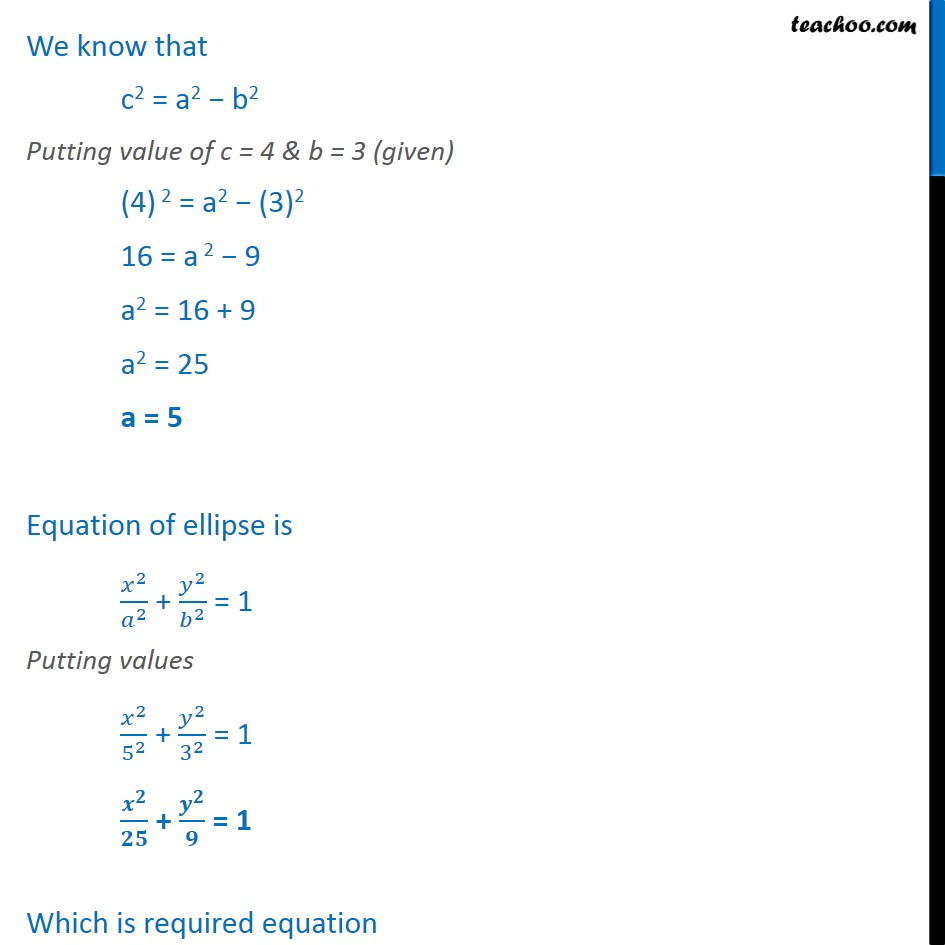1. Chapter 11 Class 11 Conic Sections
2. Serial order wise
3. Ex 11.3

Transcript

Ex 11.3, 18 Find the equation for the ellipse that satisfies the given conditions: b = 3, c = 4, centre at the origin; foci on the x axis. We need to find equation of ellipse Given b = 3, c = 4, centre at the origin & foci on the x axis. Since foci are on the x-axis So, foci are of the form (± c, 0) And major axis is along x-axis & Required equation of ellipse is ﷐﷐𝒙﷮𝟐﷯﷮﷐𝒂﷮𝟐﷯﷯ + ﷐﷐𝒚﷮𝟐﷯﷮﷐𝒃﷮𝟐﷯﷯ = 1 We know that c2 = a2 − b2 Putting value of c = 4 & b = 3 (given) (4) 2 = a2 − (3)2 16 = a 2 − 9 a2 = 16 + 9 a2 = 25 a = 5 Equation of ellipse is ﷐﷐𝑥﷮2﷯﷮﷐𝑎﷮2﷯﷯ + ﷐﷐𝑦﷮2﷯﷮﷐𝑏﷮2﷯﷯ = 1 Putting values ﷐﷐𝑥﷮2﷯﷮﷐5﷮2﷯﷯ + ﷐﷐𝑦﷮2﷯﷮﷐3﷮2﷯﷯ = 1 ﷐﷐𝒙﷮𝟐﷯﷮𝟐𝟓﷯ + ﷐﷐𝒚﷮𝟐﷯﷮𝟗﷯ = 1 Which is required equation

Ex 11.3